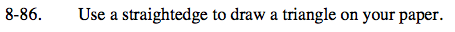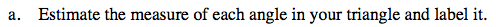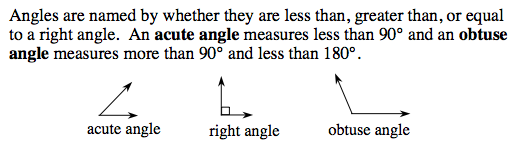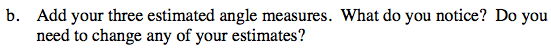### Home > CC2 > Chapter 8 > Lesson 8.3.3 > Problem8-86

8-86.
1. Use a straightedge to draw a triangle on your paper. Homework Help ✎

1. Estimate the measure of each angle in your triangle and label it.

2. Add your three estimated angle measures. What do you notice? Do you need to change any of your estimates?Refer to the Math Notes box excerpt below.
Can you estimate your angle measures by comparing them to the examples below?
Be sure your work is neat!The three angles of a triangle should add up to 180°. Do your angle estimates follow this rule?